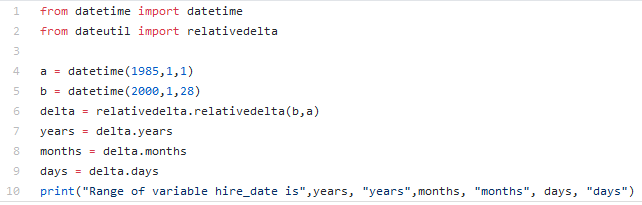{{ message }}

Instantly share code, notes, and snippets.

# amalgjose/DateDifference.py

Last active Mar 19, 2021
This is a very simple python code snippet for calculating the difference between two dates or timestamps. This will calculate the difference in terms of number of years, months, days, hours, minutes etc. For more details, refer https://amalgjose.com/2015/02/19/python-code-for-calculating-the-difference-between-two-time-stamps/
 __author__ = 'Amal G Jose' from datetime import datetime from dateutil import relativedelta ##Aug 7 1989 8:10 pm date_1 = datetime(1989, 8, 7, 20, 10) ##Dec 5 1990 5:20 am date_2 = datetime(1990, 12, 5, 5, 20) #This will find the difference between the two dates difference = relativedelta.relativedelta(date_2, date_1) years = difference.years months = difference.months days = difference.days hours = difference.hours minutes = difference.minutes print "Difference is %s year, %s months, %s days, %s hours, %s minutes " %(years, months, days, hours, minutes)

###marcaum54 commented Sep 14, 2017

 Vlew man, me salvou ;*

###moanesga commented Jun 2, 2018

 Thanks, I applied only to calculate difference in years, months and days.###andrecalais commented Sep 2, 2018

 Hi! How do I calculate the total difference in months?

###MaxMorais commented Sep 14, 2018 • edited

 @andrecalais ``````a = datetime(1985,1,1) b = datetime(2000,1,28) delta = relativedelta.relativedelta(b,a) full_months = delta.years * 12 + delta.months ``````

###oinga commented Jan 29, 2020

 Well done amalgjose! Is this snippet okay to use? Thanks!

###amalgjose commented Feb 7, 2020

 @oinga Yes you can use it.

###MohJumper commented Apr 7, 2020 • edited

 ``````# from datetime import datetime from dateutil import relativedelta # today date today = datetime.today() # year in the past yr_p = today.replace(year = 2015) difference = relativedelta.relativedelta(today, yr_p) years = difference.years months = difference.months weeks = difference.weeks days = difference.days print(f"The differnce in year: {years}, in months: {months}, in weeks: {weeks}, in days: {days}") `````` Great code, but any ideas why it does not print the months, weeks, days in my altered code?

###sathwik-teja commented Apr 16, 2020

 How to Write a Python script to display the examination schedule. Extract the exam date from user and calculate the days left for the examination from current date. output should be like this Exam date: (2016/12/30) Current date: (2016/12/15) Expected output: 15 days

###Wiper-R commented Jun 25, 2020

 How to Write a Python script to display the examination schedule. Extract the exam date from user and calculate the days left for the examination from current date. output should be like this Exam date: (2016/12/30) Current date: (2016/12/15) Expected output: 15 days ``````from datetime import date examdate = date(Year, Month, Data) currentdate = datetime.datetime.now().date() difference = relativedelta.relativedelta(today, yr_p) print(difference.days) ``````